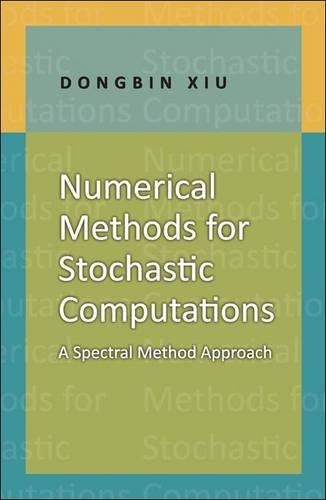•# Numerical Methods for Stochastic Computations: A Spectral Method Approach (Hardback)

(author)
£52.00
Hardback 144 Pages / Published: 13/08/2010
• We can order this

Usually dispatched within 1 week

The@ first graduate-level textbook to focus on fundamental aspects of numerical methods for stochastic computations, this book describes the class of numerical methods based on generalized polynomial chaos (gPC). These fast, efficient, and accurate methods are an extension of the classical spectral methods of high-dimensional random spaces. Designed to simulate complex systems subject to random inputs, these methods are widely used in many areas of computer science and engineering. The book introduces polynomial approximation theory and probability theory; describes the basic theory of gPC methods through numerical examples and rigorous development; details the procedure for converting stochastic equations into deterministic ones; using both the Galerkin and collocation approaches; and discusses the distinct differences and challenges arising from high-dimensional problems. The last section is devoted to the application of gPC methods to critical areas such as inverse problems and data assimilation. Ideal for use by graduate students and researchers both in the classroom and for self-study, Numerical Methods for Stochastic Computations provides the required tools for in-depth research related to stochastic computations. * The first graduate-level textbook to focus on the fundamentals of numerical methods for stochastic computations * Ideal introduction for graduate courses or self-study * Fast, efficient, and accurate numerical methods * Polynomial approximation theory and probability theory included * Basic gPC methods illustrated through examples

Publisher: Princeton University Press
ISBN: 9780691142128
Number of pages: 144
Weight: 340 g
Dimensions: 235 x 152 x 15 mm